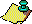Contents Next: Classical Results Interpreted Up: Continued Fractions and Chaos Previous: Introduction

# Summary of Classical Results

We begin with the classical method for finding the continued fraction representation of a number. We putequal to the integer part of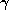, by which we mean the greatest integer less than or equal to. If the fractional part ofis not zero, we put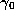equal to the fractional part of. We then invert, and putequal to the integer part of. Similarly we put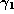equal to the fractional part, and repeat. Note that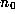may be positive, negative, or zero, but that all the subsequent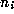will be positive, and that eachis in the interval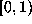. This process gives us a unique continued fraction for each starting point, and the process terminates if and only ifis rational. (For any rationalthere is one other simple continued fraction, e.g., which is only trivially different from the one generated by this algorithm.) This algorithm is related to the Euclidean algorithm for finding the greatest common divisor (gcd) of two integers k and m , in that if we use this method to find the continued fraction of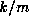, then the integer parts that arise are precisely the quotients from the Euclidean algorithm, and in fact the last nonzero remainder from the Euclidean algorithm appears as the numerator of the last nonzero fractional part. This remainder is of course the gcd of k and m. Further, this algorithm can easily be seen to terminate in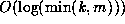operations. Click here for a note on the above paragraph, 1995.

Classically, most attention has been paid to the integers generated by this algorithm, which make up the continued fraction itself. However, Gauss was apparently the first to study the other part of this algorithm, which we present as the following map fromto, called the Gauss map  (see FIGURE 1):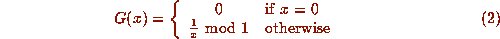We use the notation ``mod 1'' to mean taking the fractional part. In terms of the Gauss map G, our algorithm then becomes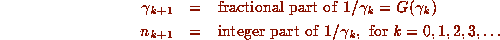(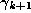is undefined if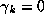) and we see that the continued fraction is generated as a byproduct of the iteration of the Gauss map. Thus we expect that any classical results on continued fractions will have implications for the dynamics of the Gauss map.

Note that the jump discontinuities occurring at(for each positive integer n) may all be removed by mapping onto the circle with the transformation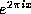. After this is done, we see that the Gauss map (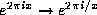) is a map of the circle onto the circle, and may be pictured on a torus, as in FIGURE 2. Click here to examine a Maple program for graphing arbitraryon the torus.

We make the following observation: if we represent a point in the intervalby its continued fraction,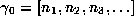, then a simple induction shows that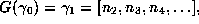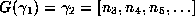,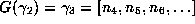, and so on. This makes a connection between the Gauss map and the shift map of symbolic dynamics . We will not explore this connection further here, but we note that the shift maps normally studied are slightly different than the Gauss map, in that here the size of the numbers in the list being ``shifted'' is not bounded. Click here for more details on the induction.

An analogy is illuminating: if we think of our space as a circular hoop with the origin at one point O on the hoop, our initial point as a dimensionless bead on the hoop, and the Gauss map as taking the bead from its current position clockwise past O at least once to its next position on the hoop, then the integersare the number of times the bead passes O on the ith iteration (in general the maximum such number is called the ``winding number'' of the map, and here this is obviously infinite), and the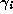are the coordinates of the bead on the hoop once it comes to rest. If the bead comes to rest close to the origin on one side, with a small, then on the next iteration it will be pushed many times around the hoop. If it comes to rest close to the origin on the other side, with aclose to 1, then it will only go past the origin once on its next iteration. We may think of the bead as being pushed around the circle, with the strength of the push being inversely proportional to the distance measured counterclockwise from the point O.Contents Next: Classical Results Interpreted Up: Continued Fractions and Chaos Previous: Introduction